# AM-compact operator

One of the most interesting classes of linear operators between Banach spaces is that of compact operators (cf. also Banach space; Linear operator; Compact operator). When the Banach spaces have some order structure (concretely: if they are Banach lattices; cf. also Banach lattice; Vector lattice; Banach function space), there are some natural classes of operators, containing the compact operators, which also have good properties and better behaviour respect to the order structure. One of these classes is that of the so-called AM-compact operators, introduced by P.S. Dodds and D.H. Fremlin in [a2] and defined as follows. Let,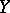be Banach lattices. A linear operator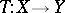is called AM-compact ifmaps order-bounded sets onto relatively compact sets.
Clearly, every compact operator is AM-compact. The converse is true ifhas a strong unit (i.e., there exists an,, such that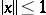implies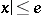). If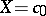or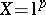, where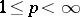, then every continuous linear operatoris AM-compact, but needs not be compact. There are important classes of operators related with the AM-compact operators. Among them are the absolute integral operators and the Dunford–Pettis operators. In particular, one has that ifandare Banach function spaces, withhaving order-continuous norm (cf. also Banach function space), then every absolute integral operator fromintois AM-compact (see [a6]). It is known (see, for instance [a3]) that ifis a Dunford–Pettis operator andhas order-continuous norm, thenis AM-compact, while ifis also an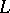-space (for instance, if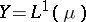), then the converse is also true.
AM-compact operators, in contrast to compact operators, have a good behaviour respect to the order in the space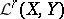of all regular operators between the Banach latticesand. Ifhas order-continuous norm, the collection of all regular AM-compact operators forms a band (cf. also Riesz space; Semi-ordered space) in; consequently, ifandis AM-compact, thenis also AM-compact.
Positive operators in Banach lattices have interesting spectral properties (see, for instance, [a5] and [a3]): the spectral radius is monotone (i.e., if, then), and the spectral radius of a positive operator is in the spectrum. In general, these two properties fail for the essential spectrum,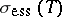, and the essential spectral radius,(cf. also Spectrum of an operator). However, B. de Pagter and A.R. Schep [a4] have established that these properties are true for AM-compact operators; they prove that ifare positive operators withandAM-compact, thenand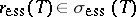. In [a1] a representation space for the essential spectrum of AM-compact operators is constructed; using this representation space the results mentioned above can be obtained with a different technique, as well as some results concerning the cyclicity of the essential spectrum for AM-compact operators.